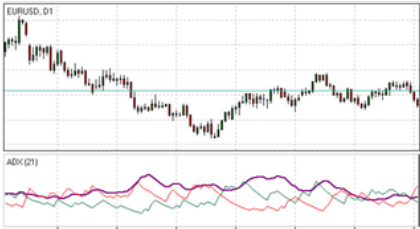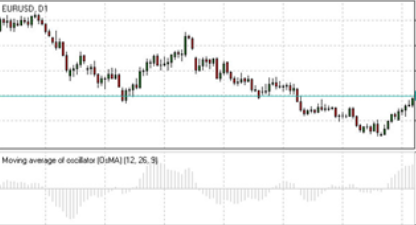# The Best Forex Trend Inidcators in Forex Trading

The Best Forex Trend Inidcators in Forex Trading – Trend indicators form the indissoluble and essential part of doing technical analysis in Forex market. They help to interpret the price movement of a currency indicating whether the price movement is strong or is likely to reverse.
Due to the trend indicators the price movement is analyzed by studying how its moving averages are trending. Either a simple moving average or an exponential moving average can be used and any period of time can be studied.

Here are presented the characteristic features and the way of calculation of the following trend indicators:
Average Directional Index (ADI), Indicator Moving Average (MA), Indicator Moving Average of Oscillator (OsMa) and Parabolic Indicator.

Average Directional Index (ADI) Indicator (How to Use ADX (Average Directional Index) in Forex Market)Average Directional Index (ADX) developed by Welles Wilder is a technical indicator which is designed to determine
trend strength and further possible price movements. This is done by comparing the difference between two

consecutive highs and the difference between lows.

Actually the direction of indicator which is marked by bold line is believed to reflect the strength of current trend:

If the ADX rises (usually surging above 25)this implies strengthening market trend.
If the ADX falls this implies that trend development is doubtful. If the value is below 20 it is a sign of neutral
trend.

• Buy signal is generated if +DI (green line) climbs above -DI (red line).
• Sell signal is generated if -DI climbs above +DI.
How to calculate Average Directional Index (ADI) Indicator?

ADX = MA [((+DI) – (-DI)) / ((+DI) + (-DI))] x 100;where:

+DI – Plus Directional Indicator;
-DI – Minus Directional Indicator.

Moving Average (MA) Indicator (How do I use Moving Average (MA) to Analysis)

Moving Average is an instrument of technical analysis which displays the average price during a certain period of time. It is used to smoothen price fluctuations and determine the strength and direction of trend.Based on the method of averaging, it’s possible to distinguish between three types of moving averages:
simple moving average (SMA),smoothed moving average (SMMA) and exponential moving average (EMA).

Moving average and price movements: (How To Use A Moving Average To Trade in Forex)
• If price crosses its rising (falling) moving average curve from below (above) a strong buy (sell) signal arises.
• If price crosses its falling (rising) moving average curve from below (above) a weak buy (sell) signal arises.
Moving average curves of different periods:
• If a rising (falling) lower-period curve crosses another rising (falling) longer-period curve from below (above) a strong buy (sell) signal arises.
• If a rising (falling) lower-period curve crosses another falling (rising) longer-period curve from below (above) a weak buy (sell) signal arises.

How to calculate Moving Average (MA)
SMA = Sum (Close (i), N) / N,
where:
Close (i) – current close price;
N – period of averaging.
EMA(t) = EMA(t-1) + (K x [Close(t) – EMA(t-1)]),
where:
t – current period;
K = 2 / (N + 1), N – period of averaging.

Moving Average of Oscillator (OsMa) – How To Use OsMa indicator To Trade in ForexMoving Average of Oscillator (OsMA) is an instrument of technical analysis which shows the difference between
the oscillator (MACD) and its moving average.
Changing the direction: (Forex osma indicator)
• If OsMA stops falling and starts rising there may be a sign of bullish reversal.
• If OsMA stops rising and starts falling there may be a sign of bearish reversal.

Crossing zero axis:

• If OsMA rises above zero a buy signal arises.
• If OsMA falls below zero a sell signal arises.

How to calculate OsMa? (Oscillator of moving average)
OsMA = MACD – Signal

Parabolic Indicator (Parabolic SAR Indicator Explained)

Parabolic indicator developed by Welles Wilder is designed to realize a number of functions:
rejecting or confirming trend direction, determining trend end, correction or flat stages, and indicating possible exit
points.The indicator is based on the principle “stop and reverse” (SAR).

Signals of trend confirmation (How to Use Parabolic Indicator in Forex)

•The indicator confirms an uptrend if it is  plotted below the price chart.

• The indicator confirms a downtrend if it is  plotted above the price chart.

Signals of exit points

• A sign of closing long positions may appear if the price falls below Parabolic line during an uptrend.
• A sign of closing short positions may appear if the price rises above Parabolic line during a downtrend.
How to calculate the Parabolic ?
P(t) = P(t-1) + AF x (EP(t-1) – P(t-1)),
where:
P(t) – current value of the indicator;
P(t-1) – value of the previous period;
AF – acceleration factor, generally rising from 0.02 to 0.2 with a step of 0.02;
EP(t-1) – Minimum/ maximum price value of the previous period.

Categories :

Tags :